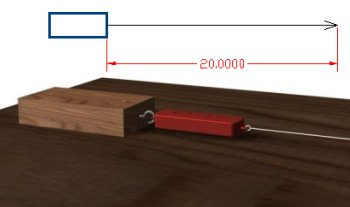Computer-Aided Design Engineering Programming Architectural Design Mechanical Design Civil Design Energy Management Electrical Design

Network
Engineer

Project
Management
 Table of ContentsWhen studying how forces act on an object, a Computer Aided Design (CAD) application program can be very useful in determining the overall magnitude and direction of the resulting force on the entity. In previous years, individuals having knowledge of this Engineering technique would find each subcomponent leg of a force that fell on the X, Y and Z axis and they could be added or subtracted based upon their direction and therefore deriving a total answer in all three axis, the resultant force acting on the body is then found. The long process to locate the sub-vectors involves the using Trigonometric functions such as Sine, Cosine and Tangent. To set up such a mathematical problem requires us to visualize the problem, so we can use the correct formula to evaluate the force distribution. Any errors in sketching, from poor hand and eye coordination ending in a sloppy illustration, or from a minor flaw in the mathematical calculation would give the designer an incorrect conclusion. In our experience, many professionals, avoid the lengthy computations and send them out to other experts. Many project managers appreciate visual design and have never really enjoyed the hand written math problems many of us are taught in school. The fast techniques we are about to show you are error free and have been used for decades. Prefix: Title Page Ch. 1: Physical Concepts within Systems of Measurement Unit 1 - Forces Ch. 2: Using CAD Software to Solve Problems Ch. 3: Forces on a 2D Plane Ch. 4: Forces in 3D Space Appendix A Unit 2 - Equilibrium Ch. 5: Free Body Diagrams Ch. 6: Equilibrium in Two Dimensions Ch. 7: Equilibrium in Three Dimensions Appendix B Unit 3 - Analyzing Structures Ch. 8: Simple Truss Ch. 9: Shear Force and Bending Diagrams Appendix C Unit 4 - Center of Gravity Ch. 10: Center of Gravity Ch. 11: Moment of Inertia Appendix D Unit 5 - Friction Ch. 12: Friction Appendix E Quiz Practice Examination (printable) Online Physics Quiz Intuitor Physics Quiz